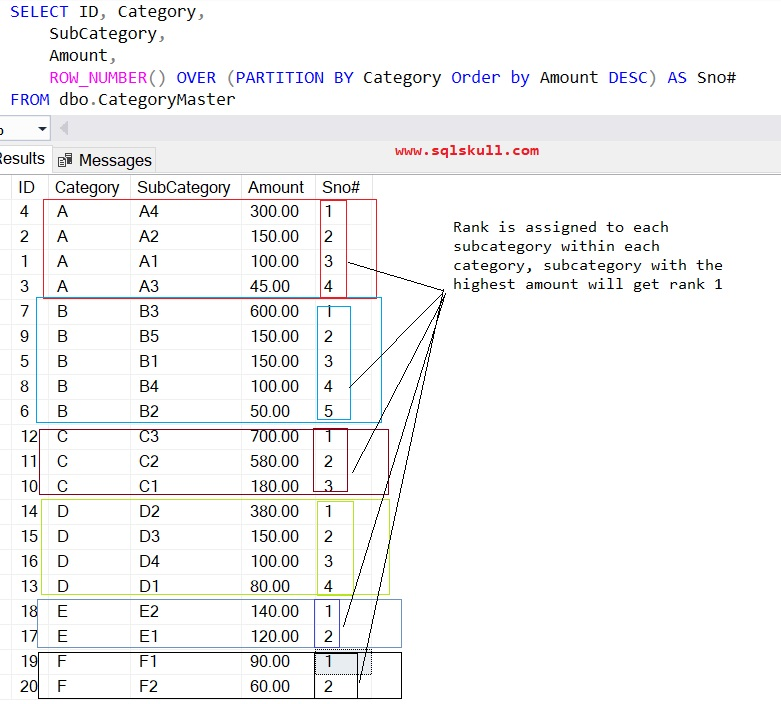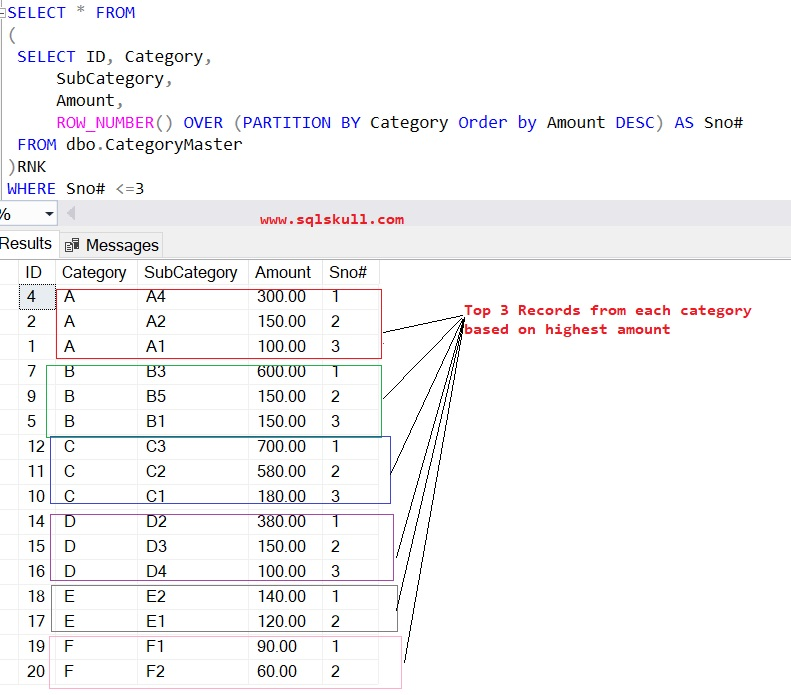Home » How to get TOP N records for each category

# How to get TOP N records for each categorySelecting a top n records for each category from any table, can be done easily using row_number function which generates a sequential integer to each row within a partition of a result set.

To understand the requirement first, for this first we will create a sample table and insert some records into table as given below.

```CREATE TABLE dbo.CategoryMaster
(ID INT IDENTITY(1,1),
Category VARCHAR(100),
Subcategory VARCHAR(100),
Amount NUMERIC(9,2))
GO

INSERT INTO dbo.CategoryMaster
(Category, Subcategory, Amount)
VALUES
('A', 'A1', 100),('A', 'A2', 150),
('A', 'A3', 45),('A', 'A4', 300),
('B', 'B1', 150),('B', 'B2', 50),
('B', 'B3', 600),('B', 'B4', 100),
('B', 'B5', 150),('C', 'C1', 180),
('C', 'C2', 580),('C', 'C3', 700),
('D', 'D1', 80),('D', 'D2', 380),
('D', 'D3', 150),('D', 'D4', 100),
('E', 'E1', 120),('E', 'E2', 140),
('F', 'F1', 90),('F', 'F2', 60)```

As you can see, now we have a table named CategoryMaster as shown below.

`SELECT * FROM dbo.CategoryMaster`As you can see, each category may have multiple records based on subcategory, now the requirement is that to get a top n records for each category from table.

Lets say you need to get top 3 records for each category that means to get a top 3 subcategory with the highest amount from each category.

To achieve this you can use SQL Server window/Ranking function

Following T-SQL uses SQL server ROW_NUMBER() Function. Which assigns a rank to each subcategory based on amount of subcategory.

```SELECT
ID,
Category,
SubCategory,
Amount,
ROW_NUMBER() OVER (PARTITION BY Category Order by Amount DESC) AS Sno#
FROM dbo.CategoryMaster```[ Also Read: SQL Server Basics Tutorial]

Lets understand the above T-SQL Code.

Row_Number function assign a rank for each subcategory by partitioning the row based on category that’s why used a Partition BY keyword on Category, and subcategory with the highest amount will be  on the top and get a rank 1 that’s why used a Order By DESC on Amount.

So far, we have assigned a rank to each subcategory within category, now we will modify above T-SQL and select only top 3 records means highest amount from each category.

Lets modify the above T-SQL as shown below.

```SELECT * FROM
(
SELECT ID, Category,
SubCategory,
Amount,
ROW_NUMBER() OVER (PARTITION BY Category Order by Amount DESC) AS Sno#
FROM dbo.CategoryMaster
)RNK
WHERE Sno# <=3```As you can see, we have converted above query as subquery and selected only those records which are having a rank value less than 3 for sno#.

Similarly if you want to see top n records for any other number lets say top10 records then you can simply replace 3 with 10 and it will return top 10 records from each category.

You will also notice that for Category E and F there are only two records because there are only two records in the table under these category so that only two records are returned.

Get top n records using CTE (common table expression)

Lets use CTE to get the top 2 records for each category from table CategoryMaster.

```;With CTE
AS
(
SELECT ID, Category,
SubCategory,
Amount,
ROW_NUMBER() OVER (PARTITION BY Category Order by Amount DESC) AS Sno#
FROM dbo.CategoryMaster
)
SELECT * FROM CTE WHERE Sno# <=2```As you can see, it returns top 2 records for each category.

SQL Server Recursive CTE

SQL Server Row_Number Function

SQL Server Interview Question & Answer

SQL Server Basics Tutorial

SSRS Tutorial